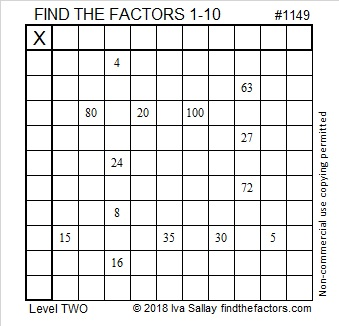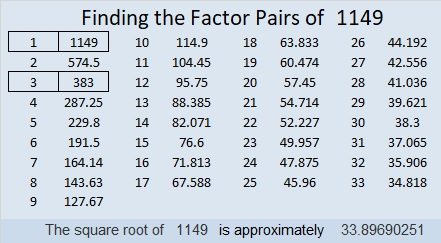# 1149 and Level 2

Look at a typical 1 – 10 multiplication table. There is only one column on it that has the numbers 63, 27, and 72. What column is that? Put that column number in the cell in the top row above those numbers and you will have done the first step in completing this puzzle.  You will need to write the numbers from 1 to 10 in both the first column and the top row to solve this puzzle. Can you find the correct places to put each number?Print the puzzles or type the solution in this excel file: 10-factors-1148-1160

Here are a few facts about the number 1149:

• 1149 is a composite number.
• Prime factorization: 1149 = 3 × 383
• The exponents in the prime factorization are 1 and 1. Adding one to each and multiplying we get (1 + 1)(1 + 1) = 2 × 2 = 4. Therefore 1149 has exactly 4 factors.
• Factors of 1149: 1, 3, 383, 1149
• Factor pairs: 1149 = 1 × 1149 or 3 × 383
• 1149 has no square factors that allow its square root to be simplified. √1149 ≈ 33.89691149 is palindrome 1D1 in BASE 28 (D is 13 base 10)
because 28² + 13(28) + 1 = 1149

This site uses Akismet to reduce spam. Learn how your comment data is processed.